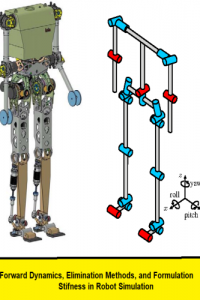اسم المؤلف
Uri M. Ascher, Dinesh K. Paiyand Benoit P. Cloutierz
التاريخ
17 ديسمبر 2019
المشاهدات
التقييم(لا توجد تقييمات)Loading...

بحث بعنوان
Forward Dynamics, Elimination Methods, and Formulation Stiffness in Robot Simulation
Uri M. Ascher, Dinesh K. Paiyand Benoit P. Cloutierz
Department of Computer Science
University of British Columbia
May 27, 1996
Abstract
The numerical simulation problem of tree-structured multibody systems,
such as robot manipulators, is usually treated as two separate problems: (i)
the forward dynamics problem for computing system accelerations, and (ii) the
numerical integration problem for advancing the state in time. The interaction of these two problems can be important and has led to new conclusions
about the overall eciency of multibody simulation algorithms (Cloutier et al.,
1995). In particular, the fastest forward dynamics methods are not necessarily the most numerically stable, and in ill-conditioned cases may slow down
popular adaptive step-size integration methods. This phenomenon is called
\formulation stiffness.”
In this paper, we first unify the derivation of both the composite rigid body
method (Walker & Orin, 1982) and the articulated-body method (Featherstone,
1983; Featherstone, 1987) as two elimination methods to solve the same linear
system, with the articulated body method taking advantage of sparsity. Then
the numerical instability phenomenon for the composite rigid body method is
explained as a cancellation error that can be avoided, or at least minimized,
when using an appropriate version of the articulated body method. Specifically,
we show that a variant of the articulated-body method is better suited to deal
with certain types of ill-conditioning than the composite rigid body method.
The unified derivation also clarifies the underlying linear algebra of forward
dynamics algorithms and is therefore of interest in its own accord.
Conclusions
While there has been considerable work in reducing the computational complexity
of algorithms for computing the forward dynamics of robot manipulators, it is only
recently that the numerical properties of these algorithms have been considered carefully. This is important since forward dynamics algorithms are typically used with
adaptive step-size numerical integrators which could interpret the poor numerical
performance of the forward dynamics as requiring much smaller step sizes. We have
shown how some of these numerical properties, in particular formulation stiffness, can
be analyzed.
We presented a unified formulation of two important methods for computing forward dynamics, viz., the composite rigid body method and the articulated body
method, as elimination methods for solving a large, sparse, linear system. The articulated body method is shown to obtain its linear time complexity by exploiting
the sparsity of the system. We analyzed the formulation stiffness of these algorithms
and showed that the poor behavior of the composite rigid body method in certain
ill-conditioned systems is due to certain cancellation errors, and this problem can be
avoided in the articulated body method. Our analysis also reveals pitfalls for implementors of the articulated body method and other forward dynamics algorithms.
A modified articulated body method with superior stability properties for general,
ill-conditioned multibody problems was proposed and demonstrated.
كلمة سر فك الضغط : books-world.net